# Electronics and Communication Engineering - Exam Questions Papers

1.
In the following non planar graph number of independent loop equations are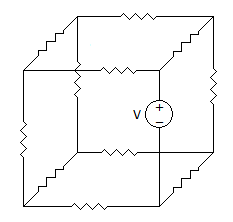8
12
7
5
Explanation:

Number of independent loop equations are given by,

L = B - N + 1

where L : No. of loop equations

B : No. of branches = 12

N : No. of nodes = 8

L = 12 - 8 + 1 = 5.

2.
A transmission line has a characteristic impedance of 50 Ω and a resistance of 0.1 Ω/m, if the line is distortion less, the attenuation constant (in Np/m) is
500
5
0.014
0.002
Explanation:

Distortion less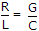a = RG and Z0 = RG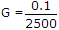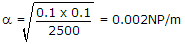.

3.
A certain 8 bit uniform quantization PCM system can accommodate a signal ranging from - 1 V to + V. The rms value of the signal is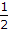V . The signal to quantization noise ratio is:
30 dB
46.91 dB
40 dB
49.92 dB
Explanation:

(SNR)dB = 1.76 + 6.02n

n = 8

Thus (SNR)dB = 1.76 + 6.02 x 8 = 49.92 dB.

4.
A rectangular waveguide, in dominant TE mode, has dimensions 10 cm x 15 cm. The cut off frequency is
10 GHz
1 GHz
15 GHz
25 GHz
Explanation:

For TE10 mode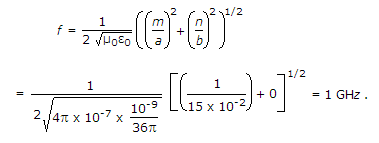5.
A radar receiver has a noise figure of 10 dB at 300 K having a bandwidth of 2.5 MHz. The minimum power it can receive is
3.45 x 10-15 W
1.38 x 10-15 W
7.5 x 10-15 W
93.15 x 10-15 W